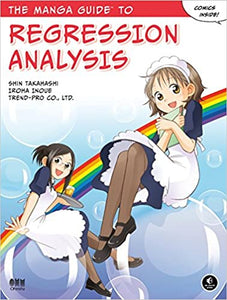Get 10% OFF your first order. Use discount code: DailyDiscount27 | FREE Shipping on orders above Rs.499/-
Get 10% OFF your first order. Use discount code: DailyDiscount27 | FREE Shipping on orders above Rs.499/-# The Manga Guide to Regression Analysis

Write a review
Regular price Rs. 1,650.00 Rs. 0.00 Unit price per

Like many people, Mui is having trouble learning regression analysis-or even staying awake in class. But with new motivation, in the form of a handsome but shy customer, and the help of her brilliant cafe'; co-worker Risa, she's determined to conquer it.

Follow along with Mui and Risa as they calculate the effect of temperature on iced tea orders, predict bakery revenues, and work out probabilities of cake sales with simple, multiple, and binomial logistic regression analysis. You'll get a refresher in basic concepts such as matrix equations, inverse functions, logarithms, and differentiation, before learning how to:

• Calculate the regression equation with sums of squares and regression coefficients
• Work out the correlation coefficient to check the accuracy of your equation
• Assess the regression analysis with hypothesis tests, analysis of variance, and confidence intervals
• Use probability density functions and odds ratios to calculate probabilities of future events
• Perform diagnostics checks to verify the validity of the analysis
• Check the goodness of fit with Chi-square and F tests
Whether you're learning regression analysis for the first time or you've just never managed to wrap your head around the topic, The Manga Guide to Regression Analysis makes mastering this tricky technique simpler, straight forward, and fun.

Liquid error: Could not find asset snippets/daily-deals.liquid